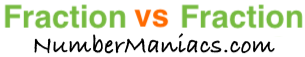4/5 vs 2/3People compare 4/5 vs 2/3 drywall, 4/5 vs 2/3 pipe, 4/5 vs 2/3 cup, 4/5 vs 2/3 ton, 4/5 vs 2/3 drill bit, 4/5 vs 2/3 poker, 4/5 vs 2/3 sump pump, 4/5 vs 2/3 garden hose, and many other items.

Is 4/5 greater than 2/3? Is 4/5 less than 2/3? What is the difference between 4/5 and 2/3? These and other questions are answered below so you can get a better perspective of 4/5 vs 2/3.

4/5 vs 2/3 Fractional Comparisons
4/5 is more than 2/3
The difference between 4/5 and 2/3 is 2/15
4/5 is 2/15 more than 2/3

4/5 vs 2/3 Decimal Comparisons
4/5 vs 2/3 as a decimal is 0.8 vs 0.667
The difference between 4/5 and 2/3 is 0.133
4/5 is 0.133 more than 2/3

4/5 vs 2/3 Percent Comparisons
4/5 vs 2/3 as a percent is 80% vs 66.7%
4/5 is 20 percent more than 2/3

Fraction vs Fraction
Submit another set of fractions that we can compare for you!

/
vs
/

The math behind the numbers
Calculations on this page are rounded to the nearest thousandth if necessary. Below is the math we used to analyze 4/5 vs 2/3:

To compare the size of 4/5 vs 2/3, we simply subtracted 2/3 from 4/5:

4/5 - 2/3 = |2/15|

To find 4/5 vs 2/3 as a decimal, we divided the numerator by the denominator for each fraction.

4 ÷ 5 = 0.8
2 ÷ 3 = 0.667

To find 4/5 vs 2/3 as a percent, we divided the numerator by the denominator and then multiplied the quotient by 100 for each fraction.

(4 ÷ 5) x 100 = 80%
(2 ÷ 3) x 100 = 66.7%

We found that 4/5 is 20 percent more than 2/3 by dividing the difference between the two fractions by the second fraction and then multiplied it by 100:

((2/15)/(2/3))×100 = 20%

That's it folks. We hope this page gave you a better understanding of 4/5 vs 2/3 with our explanations and conclusions.

4/5 vs 2/4
Here are the next two fractions we have compared and analyzed.